• 小波与小波包、小波包分解与信号重构、小波包能量特征提取 (Matlab 程序详解） -----暨 小波包分解后解决频率大小分布重新排列问题 本人当前对小波理解不是很深入，通过翻阅网络他人博客，进行汇总总结，重新...
        小波与小波包、小波包分解与信号重构、小波包能量特征提取   (Matlab 程序详解）

-----暨 小波包分解后解决频率大小分布重新排列问题

本人当前对小波理解不是很深入，通过翻阅网络他人博客，进行汇总总结，重新调试Matlab代码，实现对小波与小波包、小波包分解与信号重构、小波包能量特征提取，供大家参考，后续将继续更新！

本人在分析信号的过程中发现，按照网上所述的小波包分解方法理解，获取每层节点重构后信号频率并不是按照（n,0）、(n,1)...顺序依次由小到大排列的，经过进一步分析研究后发现，需要对节点进行重排序，具体操作见本文分析。

1.小波与小波包区别

工程应用中经常需要对一些非平稳信号进行，小波分析和小波包分析适合对非平稳信号分析，相比较小波分析，利用小波包分析可以对信号分析更加精细，小波包分析可以将时频平面划分的更为细致，对信号的高频部分的分辨率要好于小波分析，可以根据信号的特征，自适应的选择最佳小波基函数，比便更好的对信号进行分析，所以小波包分析应用更加广泛。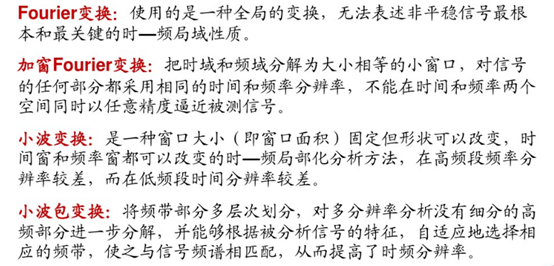①小波分解

小波变换只对信号的低频部分做进一步分解，而对高频部分也即信号的细节部分不再继续分解，所以小波变换能够很好地表征一大类以低频信息为主要成分的信号，不能很好地分解和表示包含大量细节信息（细小边缘或纹理）的信号，如非平稳机械振动信号、遥感图象、地震信号和生物医学信号等。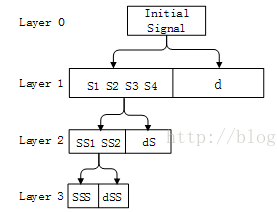②小波包分解

小波包变换既可以对低频部分信号进行分解，也可以对高频部分进行分解，而且这种分解既无冗余，也无疏漏，所以对包含大量中、高频信息的信号能够进行更好的时频局部化分析。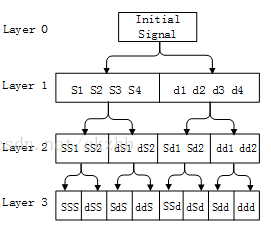2.小波包——小波包树与时频图

小波包树解读：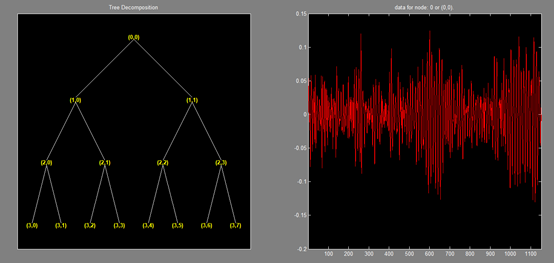以上即是小波包树，其中节点的命名规则是从（1，0）开始，叫1号， （1，1）是2号………依此类推，（3，0）是7号，（3，7）是14号。 每个节点都有对应的小波包系数，这个系数决定了频率的大小，也就是说频率信息已经有了，但是时域信息在哪里呢？ 那就是 order。  这个order就是这些节点的顺序，也就是频率的顺序。

Matlab实例：

采样频率为1024Hz，采样时间是1秒，有一个信号s是由频率100和200Hz的正弦波混合的，我们用小波包来分解。

clear all
clc
fs=1024;  %采样频率
f1=100;   %信号的第一个频率
f2=300;   %信号第二个频率
t=0:1/fs:1;
s=sin(2*pi*f1*t)+sin(2*pi*f2*t);  %生成混合信号
[tt]=wpdec(s,3,'dmey');  %小波包分解，3代表分解3层，'dmey'使用meyr小波
plot(tt)               %画小波包树图
wpviewcf(tt,1);        %画出时间频率图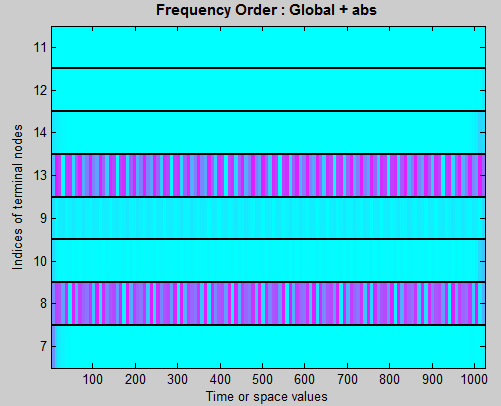主要解释：

x轴，就是1024个点，对应1秒，每个点就代表1/1024秒。

y轴，显示的数字对应于小波包树中的节点，从下面开始，顺序是 7号节点，8号，10号，9号，，，，11号节点，这个顺序是这么排列的，这是小波包自动排列的。然后，y轴是频率啊，怎么不是 100Hz和300Hz呢？我们的采样频率是1024Hz，根据采样定理，奈奎斯特采样频率是512Hz，我们分解了3层，最后一层就是 2^3=8个频率段，每个频率段的频率区间是 512/8=64Hz,看图颜色重的地方一个是在8那里，一个在13那里，8是第二段，也就是 65-128Hz之间，13是第五段，也就是257-320Hz之间。这样就说通了，正好这个原始信号只有两个频率段，一个100一个300。如果我们不是分解了3层，而是更多层，那么每个频率段包含的频率也就越窄，图上有颜色的地方也会更细，也就是说更精细了，由于原始信号的频率在整个1秒钟内都没有改变，所以有颜色的地方是一个横线。（引用：http://www.cnblogs.com/welen/articles/5667217.html ）

3.小波包-----小波包分解系数

在数值分析中，我们学过内积，内积的物理含义：两个图形的相似性，若两个图形完全正交，则内积为0，若两个图形完全一样，则系数为1（相对值）。小波变换的实质是：原信号与小波基函数的相似性。小波系数就是小波基函数与原信号相似的系数。

连续小波变换：小波函数与原信号对应点相乘，再相加，得到对应点的小波变换系数，平移小波基函数，再计算小波函数与原信号对应点相乘，再相加，这样就得到一系列的小波系数。对于离散小波变换（由于很多小波函数不是正交函数，因此需要一个尺度函数）所以，原信号函数可以分解成尺度函数和小波函数的线性组合，在这个函数中，尺度函数产生低频部分，小波函数产生高频部分。

4.小波包-----信号分解与重构（方法1）

该方法可以实现对任意节点系数选择进行组合重构。

例1

有一个信号，变量名为wave，随便找一个信号load进来就行了。

t=wpdec(wave,3,'dmey');
t2 = wpjoin(t,[3;4;5;6]);
sNod = read(t,'sizes',[3,4,5,6]);
cfs3  = zeros(sNod(1,:));
cfs4  = zeros(sNod(2,:));
cfs5  = zeros(sNod(3,:));
cfs6  = zeros(sNod(4,:));
t3 = write(t2,'cfs',3,cfs3,'cfs',4,cfs4,'cfs',5,cfs5,'cfs',6,cfs6);
wave2=wprec(t3);


第一行：将wave 用 dmey小波进行3层小波包分解，获得一个小波包树 t

第二行：将小波包树的第二行的四个节点收起来，也就是让第二行的节点变为树的最底层节点。因为第一行中小波包树的节点个数是第一层2个，第二层4个，第三层8个。现在t2就是将第三层的节点再聚合回第二层。

第三行：读取第二层四个节点系数的size

第四~七行：将所有四个节点的小波包系数变为0

第八行：将四个节点的系数重组到t3小波树中。

第九行：对t3小波树进行重构，获得信号wave2

可以预见，因为我们把小波树的节点系数都变为0了，所以信号也就全为0了。所以wave2是一个0向量。读者可以自行plot一下wave和wave2看看。进一步，如果我们只聚合第二层中的某几个节点，比如 4和5，即将第三行到第八行中节点 3 和节点 6的语句删除或修改，那么意思就是将 4  5节点的系数变为0，那么wave2肯定就不是0向量了。

例2

t=wpdec(wave,3,'dmey');
t2 = wpjoin(t,[3;4;5;6]);
cfs3=wpcoef(t,3);
cfs4=wpcoef(t,4);
cfs5=wpcoef(t,5);
cfs6=wpcoef(t,6);
t3 = write(t2,'cfs',3,cfs3,'cfs',4,cfs4,'cfs',5,cfs5,'cfs',6,cfs6);
wave2=wprec(t3);


解释：

第一行：将wave 用 dmey小波进行3层小波包分解，获得一个小波包树 t

第二行：将小波包树的第二行的四个节点收起来，也就是让第二行的节点变为树的最底层节点。

第三~六行：获取四个节点的小波包系数 （小波包系数就是一个一维向量）

第七行：将四个节点的系数重组到t3小波树中

第八行：对t3小波树进行重构，获得信号wave2

可以看出，该例子就是对一个小波包展开了，又原封不动的装回去了，所以说 wave2和wave是一样的。

注意，wpjoin命令在这里是必要的，因为write函数只能将最底层的节点写进去。也就是说，如果我们将第三层的小波包系数进行修改的话，就不用wpjoin了，具体可以看例3

例3

t=wpdec(wave,3,'dmey');
cfs7=wpcoef(t,7);
cfs8=wpcoef(t,8);
cfs9=wpcoef(t,9);
cfs10=wpcoef(t,10);
cfs11=wpcoef(t,11);
cfs12=wpcoef(t,12);
cfs13=wpcoef(t,13);
cfs14=wpcoef(t,14);
t3=write(t,'cfs',7,cfs7,'cfs',8,cfs8,'cfs',9,cfs9,'cfs',10,cfs10,'cfs',11,cfs11,'cfs',...
12,cfs12,'cfs',13,cfs13,'cfs',14,cfs14);
y=wprec(t3);


该例子也是对一个小波包展开了，又原封不动的装回去了，只不过这次是直接对第三层节点进行的。

5.小波包-----信号分解与重构（方法2）

该方法只能对某一节点信号系数分别进行重构，不能实现多个节点系数组合进行重构.

接下来进行举例说明：

x_input=x_train(:,1,1);                  %输入数据
plot(x_input);title('输入信号时域图像')   %绘制输入信号时域图像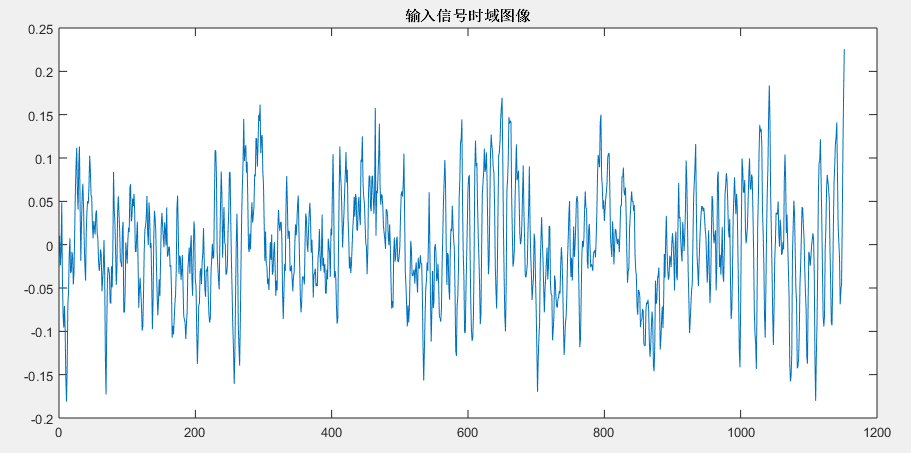%%   查看频谱范围
x=x_input;
fs=128;
N=length(x); %采样点个数
signalFFT=abs(fft(x,N));%真实的幅值
Y=2*signalFFT/N;
f=(0:N/2)*(fs/N);
figure;plot(f,Y(1:N/2+1));
ylabel('amp'); xlabel('frequency');title('输入信号的频谱');grid on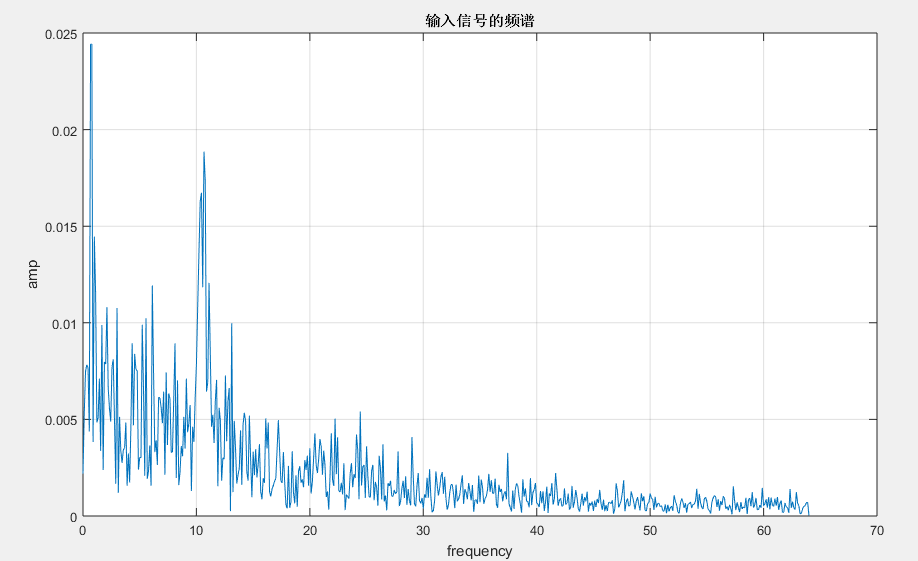接下来进行4层小波包分解

wpt=wpdec(x_input,3,'dmey');        %进行3层小波包分解
plot(wpt);                          %绘制小波包树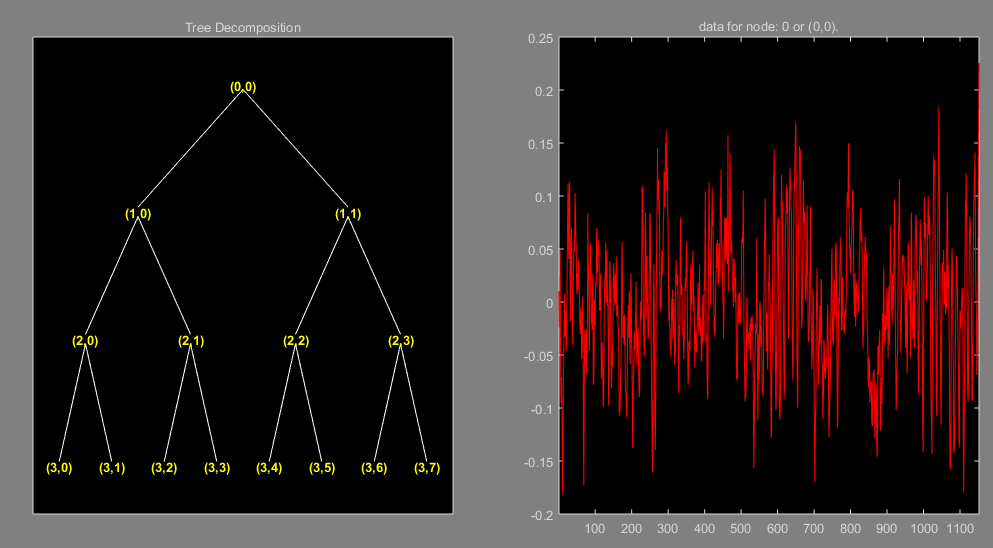接下来，我们查看第3层8个节点的频谱分布（我的输入信号采样频率是128，按照采样定理小波包分解根节点（0，0）处的频率应该为0-64Hz，按照这个计算（3，0）节点为0-8Hz，后面依次以8Hz为一个段递增）

首先展示最初的重构方法（频率排布顺序混乱，常见理解错误）

for i=0:7
rex3(:,i+1)=wprcoef(wpt,[3 i]);  %实现对节点小波节点进行重构
end

figure;                          %绘制第3层各个节点分别重构后信号的频谱
for i=0:7
subplot(2,4,i+1);
x_sign=rex3(:,i+1);
N=length(x_sign); %采样点个数
signalFFT=abs(fft(x_sign,N));%真实的幅值
Y=2*signalFFT/N;
f=(0:N/2)*(fs/N);
plot(f,Y(1:N/2+1));
ylabel('amp'); xlabel('frequency');grid on
axis([0 50 0 0.03]); title(['小波包第3层',num2str(i),'节点信号频谱']);
end

绘制完后你会发现频谱分布并不是按照之前理解的顺序依次递增排列，如下所示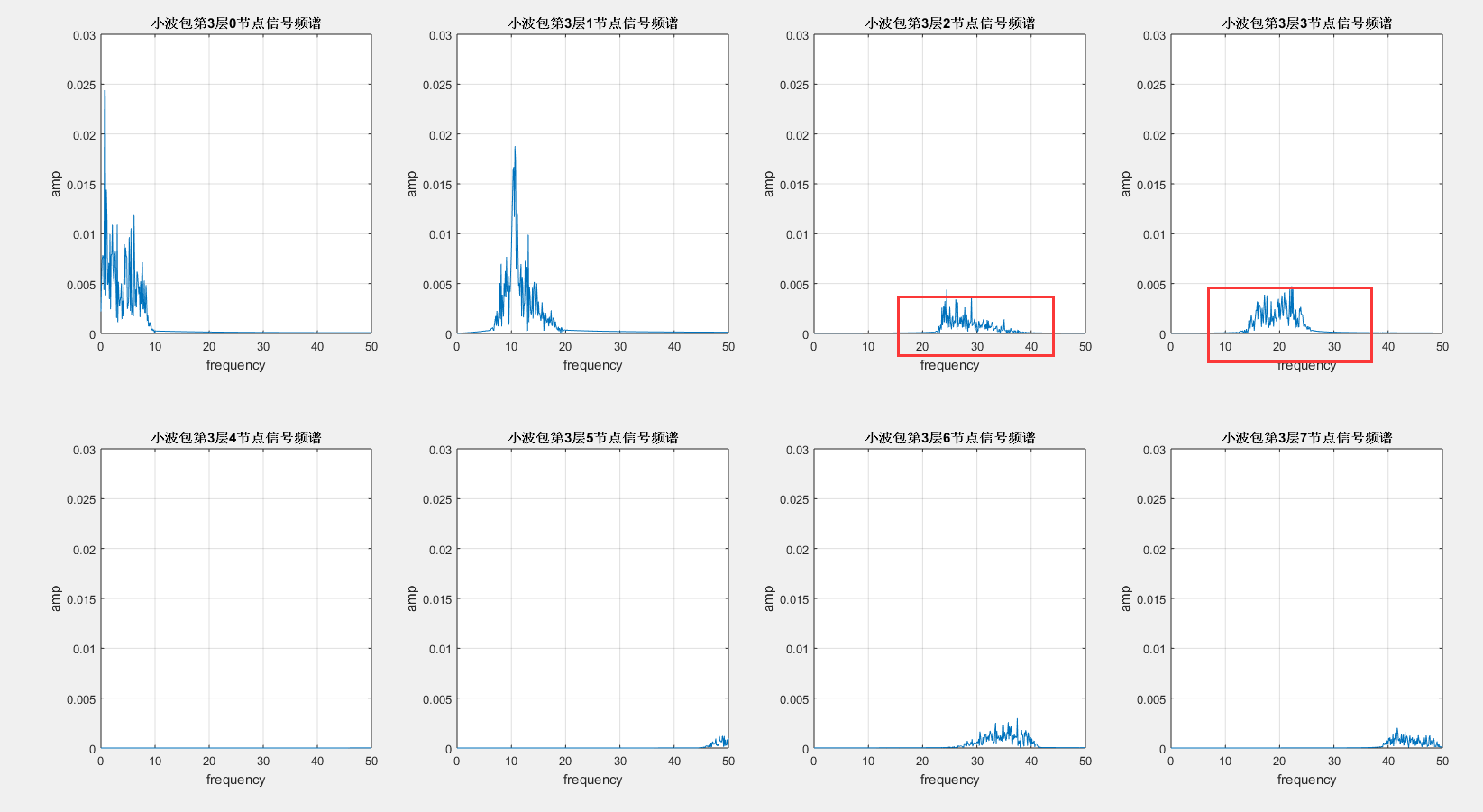那么问题出在哪里呢？经过仔细深入验证后发现问题出在小波包节点的频谱划分“不是严格按照上述理解的顺序排列的”（可能是一种格雷编码或者其他），要解决这个问题我们需要对节点顺序进行重新编码排序。参考这里https://www.ilovematlab.cn/thread-122226-1-1.html?tdsourcetag=s_pctim_aiomsg

nodes=[7;8;9;10;11;12;13;14];   %第3层的节点号
ord=wpfrqord(nodes);  %小波包系数重排，ord是重排后小波包系数索引构成的矩阵　如3层分解的[1;2;4;3;7;8;6;5]
nodes_ord=nodes(ord); %重排后的小波系数

注意：节点的命名规则是从（1，0）开始，叫1号， （1，1）是2号………依此类推，（3，0）是7号，（3，7）是14号。

那么我们来看看经过上面这段重排序运行后nodes_ord中顺序是什么？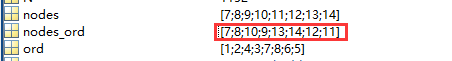接下来我们再绘制一下第三层8个节点重构信号的频谱如下

for i=1:8
rex3(:,i)=wprcoef(wpt,nodes_ord(i));  %实现对节点小波节点进行重构
end

figure;                         %绘制第3层各个节点分别重构后信号的频谱
for i=0:7
subplot(2,4,i+1);
x_sign= rex3(:,i+1);
N=length(x_sign); %采样点个数
signalFFT=abs(fft(x_sign,N));%真实的幅值
Y=2*signalFFT/N;
f=(0:N/2)*(fs/N);
plot(f,Y(1:N/2+1));
ylabel('amp'); xlabel('frequency');grid on
axis([0 50 0 0.03]); title(['小波包第3层',num2str(i),'节点信号频谱']);
end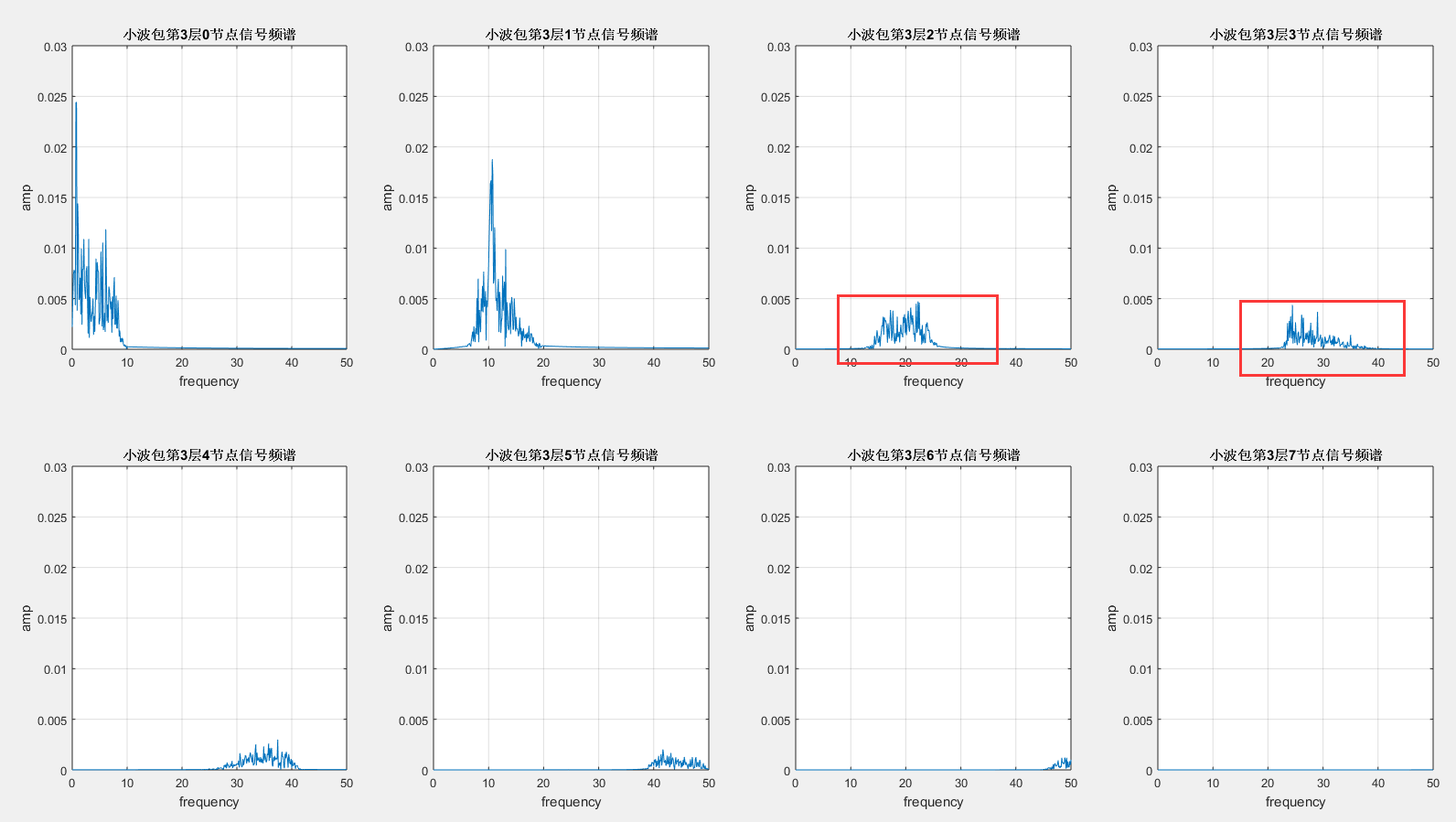到这里为止不知道大家有没有明白我要表达的意思，如果没有明白可以再反复看理解，或者自己进行分解后观察每个节点的频谱后或许就理解了。

6.小波包分解------能量特征提取（方法1）

接下来绘制第3层各个频段的能量占比

%% wavelet packet coefficients. 求取小波包分解的各个节点的小波包系数
cfs3_0=wpcoef(wpt,nodes_ord(1));  %对重排序后第3层0节点的小波包系数0-8Hz
cfs3_1=wpcoef(wpt,nodes_ord(2));  %对重排序后第3层0节点的小波包系数8-16Hz
cfs3_2=wpcoef(wpt,nodes_ord(3));  %对重排序后第3层0节点的小波包系数16-24Hz
cfs3_3=wpcoef(wpt,nodes_ord(4));  %对重排序后第3层0节点的小波包系数24-32Hz
cfs3_4=wpcoef(wpt,nodes_ord(5));  %对重排序后第3层0节点的小波包系数32-40Hz
cfs3_5=wpcoef(wpt,nodes_ord(6));  %对重排序后第3层0节点的小波包系数40-48Hz
cfs3_6=wpcoef(wpt,nodes_ord(7));  %对重排序后第3层0节点的小波包系数48-56Hz
cfs3_7=wpcoef(wpt,nodes_ord(8));  %对重排序后第3层0节点的小波包系数56-64Hz

E_cfs3_0=norm(cfs3_0,2)^2;  %% 1-范数：就是norm(...,1)，即各元素绝对值之和；2-范数：就是norm(...,2)，即各元素平方和开根号；
E_cfs3_1=norm(cfs3_1,2)^2;
E_cfs3_2=norm(cfs3_2,2)^2;
E_cfs3_3=norm(cfs3_3,2)^2;
E_cfs3_4=norm(cfs3_4,2)^2;
E_cfs3_5=norm(cfs3_5,2)^2;
E_cfs3_6=norm(cfs3_6,2)^2;
E_cfs3_7=norm(cfs3_7,2)^2;
E_total=E_cfs3_0+E_cfs3_1+E_cfs3_2+E_cfs3_3+E_cfs3_4+E_cfs3_5+E_cfs3_6+E_cfs3_7;

p_node(0)= 100*E_cfs3_0/E_total;           % 求得每个节点的占比
p_node(2)= 100*E_cfs3_1/E_total;           % 求得每个节点的占比
p_node(3)= 100*E_cfs3_2/E_total;           % 求得每个节点的占比
p_node(4)= 100*E_cfs3_3/E_total;           % 求得每个节点的占比
p_node(5)= 100*E_cfs3_4/E_total;           % 求得每个节点的占比
p_node(6)= 100*E_cfs3_5/E_total;           % 求得每个节点的占比
p_node(7)= 100*E_cfs3_6/E_total;           % 求得每个节点的占比
p_node(8)= 100*E_cfs3_7/E_total;           % 求得每个节点的占比

figure;
x=1:8;
bar(x,p_node);
title('各个频段能量所占的比例');
xlabel('频率 Hz');
ylabel('能量百分比/%');
for j=1:8
text(x(j),p_node(j),num2str(p_node(j),'%0.2f'),...
'HorizontalAlignment','center',...
'VerticalAlignment','bottom')
end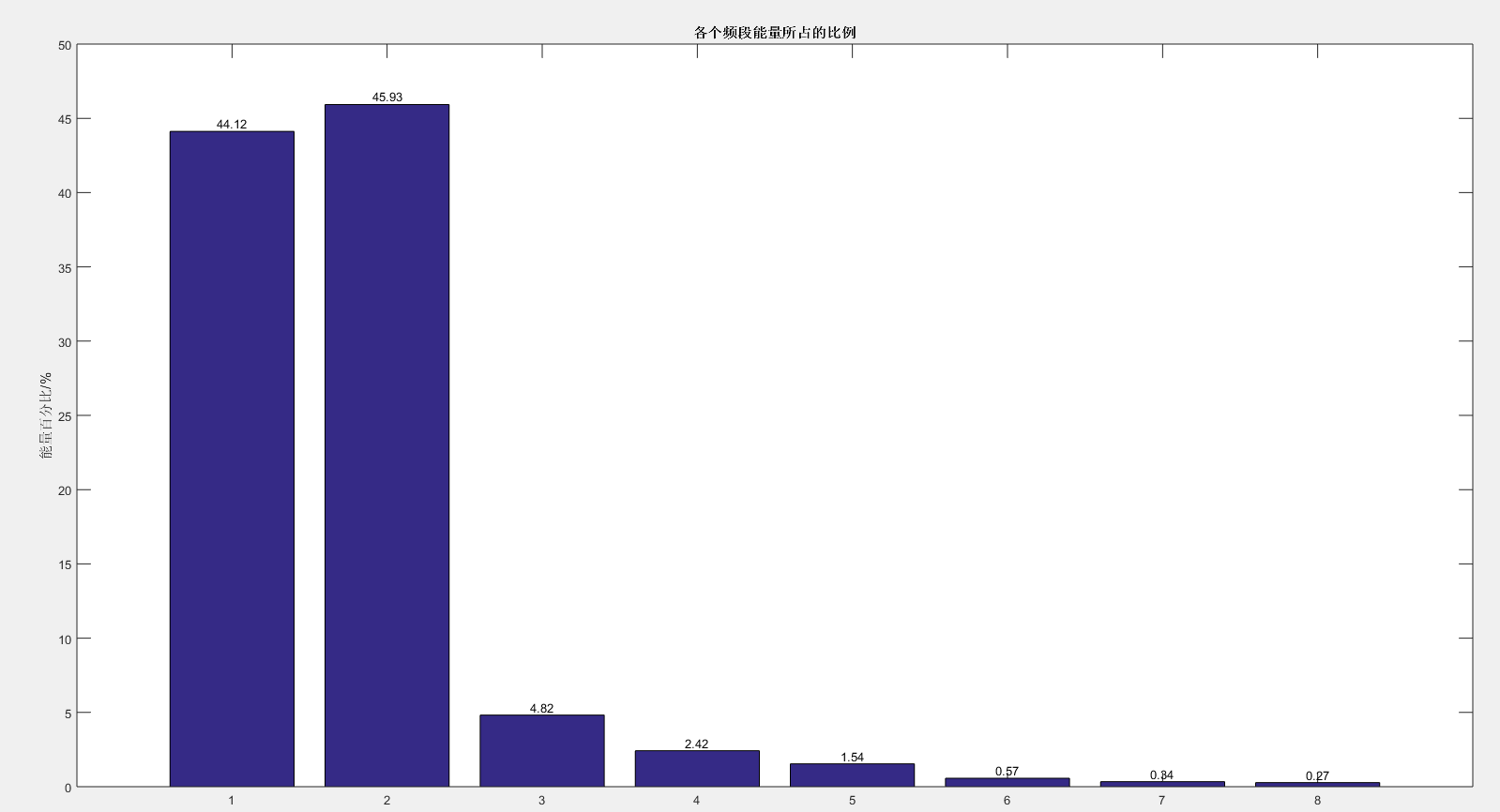7.小波包分解------能量特征提取（方法2）

直接运行matlab自带函数，如下

E = wenergy(wpt);   %该函数只能对最后一层（底层）节点进行能量提取

（引用：https://blog.csdn.net/it_beecoder/article/details/78668273）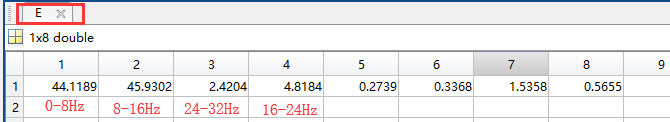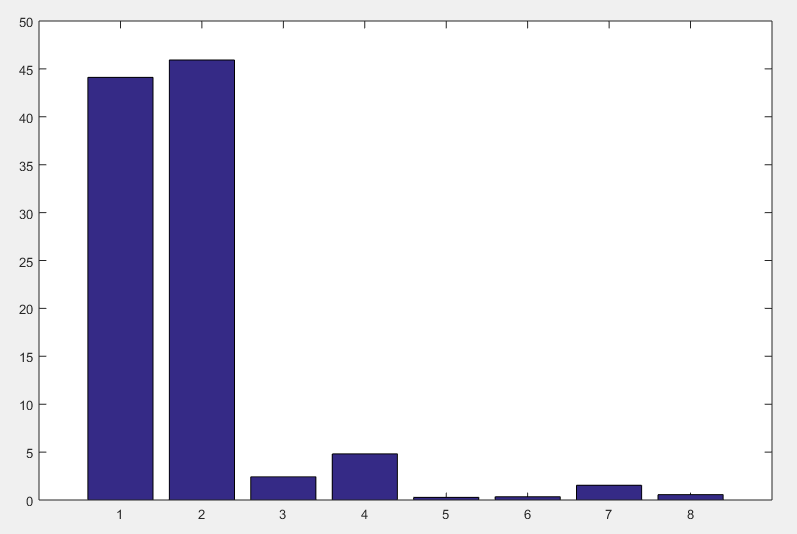这里对比一下，和方法1得到的图形基本上是一致的，不同之处在于排列顺序变了，方法2中的顺序是按照小波包分解函数自动排列顺序，方法1经过重排序后为按照频率段递增顺序依次排列顺序的

%% 绘制重构前各个频段小波包系数
figure(1);
subplot(4,1,1);
plot(x_input);
title('原始信号');
subplot(4,1,2);
plot(cfs3_0);
title(['层数 ',num2str(3) '  节点 0的小波0-8Hz',' 系数'])
subplot(4,1,3);
plot(cfs3_1);
title(['层数 ',num2str(3) '  节点 1的小波8-16Hz',' 系数'])
subplot(4,1,4);
plot(cfs3_2);
title(['层数 ',num2str(3) '  节点 2的小波16-24Hz',' 系数'])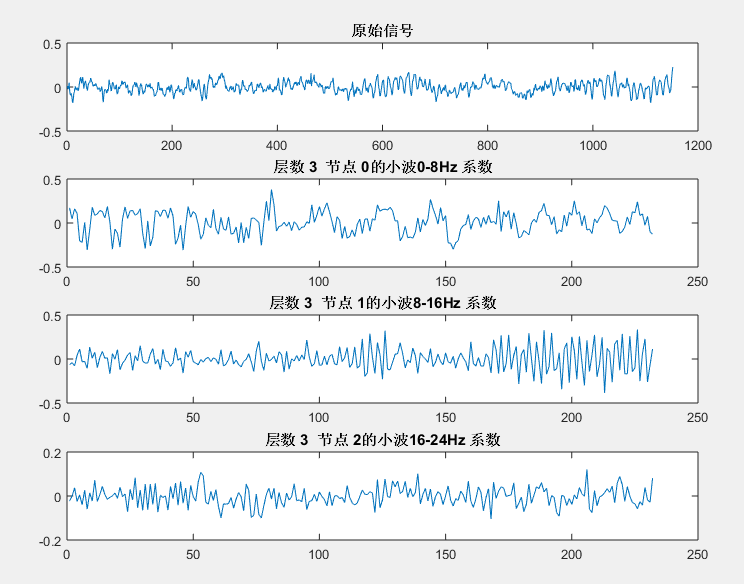%% 绘制重构后时域各个特征频段的图形
figure(3);
subplot(3,1,1);
plot(rex3(:,1));
title('重构后0-8Hz频段信号');
subplot(3,1,2);
plot(rex3(:,2));
title('重构后8-16Hz频段信号')
subplot(3,1,3);
plot(rex3(:,3));
title('重构后16-24Hz频段信号');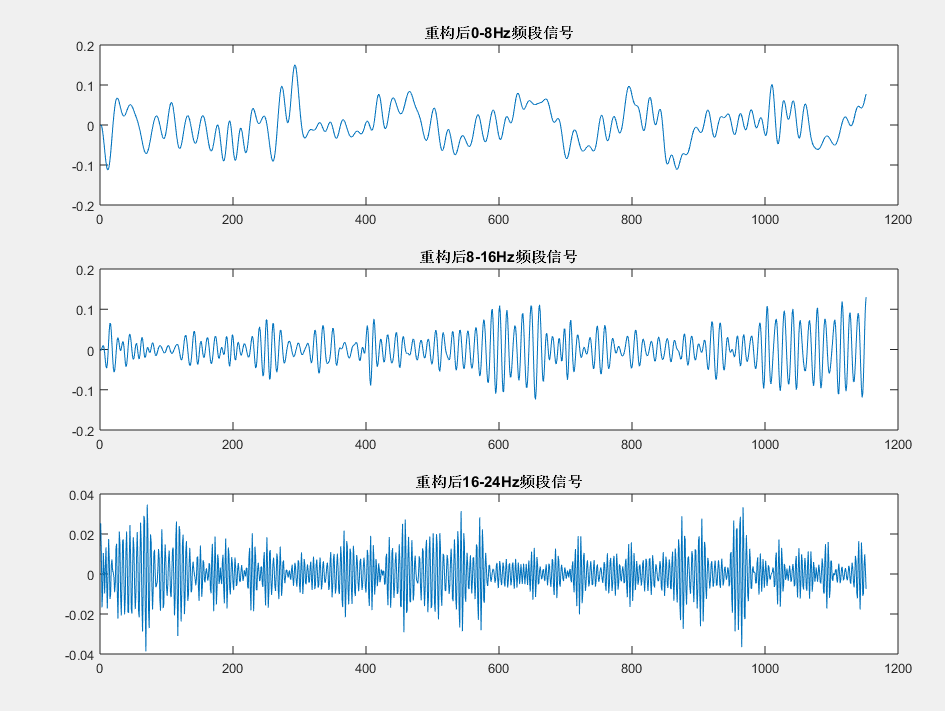以上过程完整代码

clear all;

x_input=x_train(:,1,1);           %输入数据
plot(x_input);title('输入信号时域图像')  %绘制输入信号时域图像

x=x_input;        %   查看频谱范围
fs=128;
N=length(x); %采样点个数
signalFFT=abs(fft(x,N));%真实的幅值
Y=2*signalFFT/N;
f=(0:N/2)*(fs/N);
figure;plot(f,Y(1:N/2+1));
ylabel('amp'); xlabel('frequency');title('输入信号的频谱');grid on

wpt=wpdec(x_input,3,'dmey');      %进行4层小波包分解
plot(wpt);                        %绘制小波包树

%% 实现对节点顺序按照频率递增进行重排序
% nodes=get(wpt,'tn');  %小波包分解系数　为什么ｎｏｄｅｓ是[7;8;9;10;11;12;13;14]
% N_cfs=length(nodes);  %小波包系数个数
nodes=[7;8;9;10;11;12;13;14];
ord=wpfrqord(nodes);  %小波包系数重排，ord是重排后小波包系数索引构成的矩阵　如3层分解的[1;2;4;3;7;8;6;5]
nodes_ord=nodes(ord); %重排后的小波系数

%% 实现对节点小波节点进行重构
for i=1:8
rex3(:,i)=wprcoef(wpt,nodes_ord(i));
end

%% 绘制第3层各个节点分别重构后信号的频谱
figure;
for i=0:7
subplot(2,4,i+1);
x_sign= rex3(:,i+1);
N=length(x_sign); %采样点个数
signalFFT=abs(fft(x_sign,N));%真实的幅值
Y=2*signalFFT/N;
f=(0:N/2)*(fs/N);
plot(f,Y(1:N/2+1));
ylabel('amp'); xlabel('frequency');grid on
axis([0 50 0 0.03]); title(['小波包第3层',num2str(i),'节点信号频谱']);
end

%% wavelet packet coefficients. 求取小波包分解的各个频段的小波包系数
cfs3_0=wpcoef(wpt,nodes_ord(1));  %对重排序后第3层0节点的小波包系数0-8Hz
cfs3_1=wpcoef(wpt,nodes_ord(2));  %对重排序后第3层0节点的小波包系数8-16Hz
cfs3_2=wpcoef(wpt,nodes_ord(3));  %对重排序后第3层0节点的小波包系数16-24Hz
cfs3_3=wpcoef(wpt,nodes_ord(4));  %对重排序后第3层0节点的小波包系数24-32Hz
cfs3_4=wpcoef(wpt,nodes_ord(5));  %对重排序后第3层0节点的小波包系数32-40Hz
cfs3_5=wpcoef(wpt,nodes_ord(6));  %对重排序后第3层0节点的小波包系数40-48Hz
cfs3_6=wpcoef(wpt,nodes_ord(7));  %对重排序后第3层0节点的小波包系数48-56Hz
cfs3_7=wpcoef(wpt,nodes_ord(8));  %对重排序后第3层0节点的小波包系数56-64Hz
%% 求取小波包分解的各个频段的能量
E_cfs3_0=norm(cfs3_0,2)^2;  %% 1-范数：就是norm(...,1)，即各元素绝对值之和；2-范数：就是norm(...,2)，即各元素平方和开根号；
E_cfs3_1=norm(cfs3_1,2)^2;
E_cfs3_2=norm(cfs3_2,2)^2;
E_cfs3_3=norm(cfs3_3,2)^2;
E_cfs3_4=norm(cfs3_4,2)^2;
E_cfs3_5=norm(cfs3_5,2)^2;
E_cfs3_6=norm(cfs3_6,2)^2;
E_cfs3_7=norm(cfs3_7,2)^2;
E_total=E_cfs3_0+E_cfs3_1+E_cfs3_2+E_cfs3_3+E_cfs3_4+E_cfs3_5+E_cfs3_6+E_cfs3_7;

p_node(0)= 100*E_cfs3_0/E_total;           % 求得每个节点的占比
p_node(2)= 100*E_cfs3_1/E_total;           % 求得每个节点的占比
p_node(3)= 100*E_cfs3_2/E_total;           % 求得每个节点的占比
p_node(4)= 100*E_cfs3_3/E_total;           % 求得每个节点的占比
p_node(5)= 100*E_cfs3_4/E_total;           % 求得每个节点的占比
p_node(6)= 100*E_cfs3_5/E_total;           % 求得每个节点的占比
p_node(7)= 100*E_cfs3_6/E_total;           % 求得每个节点的占比
p_node(8)= 100*E_cfs3_7/E_total;           % 求得每个节点的占比

figure;
x=1:8;
bar(x,p_node);
title('各个频段能量所占的比例');
xlabel('频率 Hz');
ylabel('能量百分比/%');
for j=1:8
text(x(j),p_node(j),num2str(p_node(j),'%0.2f'),...
'HorizontalAlignment','center',...
'VerticalAlignment','bottom')
end
% E = wenergy(wpt);       %求取各个节点能量

%% 绘制重构前各个特征频段小波包系数的图形
figure(1);
subplot(4,1,1);
plot(x_input);
title('原始信号');
subplot(4,1,2);
plot(cfs3_0);
title(['层数 ',num2str(3) '  节点 0的小波0-8Hz',' 系数'])
subplot(4,1,3);
plot(cfs3_1);
title(['层数 ',num2str(3) '  节点 1的小波8-16Hz',' 系数'])
subplot(4,1,4);
plot(cfs3_2);
title(['层数 ',num2str(3) '  节点 2的小波16-24Hz',' 系数'])

%% 绘制重构后时域各个特征频段的图形
figure(3);
subplot(3,1,1);
plot(rex3(:,1));
title('重构后0-8Hz频段信号');
subplot(3,1,2);
plot(rex3(:,2));
title('重构后8-16Hz频段信号')
subplot(3,1,3);
plot(rex3(:,3));
title('重构后16-24Hz频段信号');



8.小波----常见基函数

与标准的傅里叶变换相比，小波分析中使用到的小波函数具有不唯一性，即小波函数 具有多样性。小波分析在工程应用中，一个十分重要的问题就是最优小波基的选择问题，因为用不同的小波基分析同一个问题会产生不同的结果。目前我们主要是通过用小波分析方法处理信号的结果与理论结果的误差来判定小波基的好坏，由此决定小波基。

常用小波基有Haar小波、Daubechies(dbN)小波、Mexican Hat(mexh)小波、Morlet小波、Meyer小波等。

1.Haar小波Haar函数是小波分析中最早用到的一个具有紧支撑的正交小波函数，也是最简单的一个小波函数，它是支撑域在范围内的单个矩形波。Haar函数的定义如下：Haar小波在时域上是不连续的，所以作为基本小波性能不是特别好。但它也有自己的优点:计算简单。不但与正交，而且与自己的整数位移正交，因此，在的多分辨率系统中，Haar小波构成一组最简单的正交归一的小波族。

  [phi,g1,xval] = wavefun('haar',20);
subplot(2,1,1);
plot(xval,g1,'LineWidth',2);
xlabel('t')；
title('haar 时域');
g2=fft(g1);
g3=abs(g2);
subplot(2,1,2);
plot(g3,'LineWidth',2);
xlabel('f')title('haar 频域')；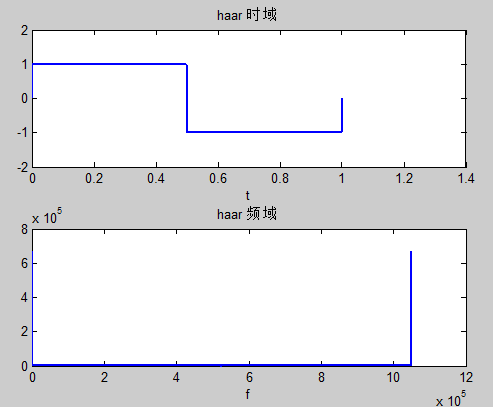2.Daubechies(dbN)小波Daubechies小波是世界著名的小波分析学者Inrid·Daubechies构造的小波函数，简写为dbN，N是小波的阶数。小波和尺度函数中的支撑区为的消失矩为。除（Harr小波）外，dbN不具有对称性（即非线性相位）。除（Harr小波）外，dbN没有明确的表达式，但转换函数h的平方模是明确的:令，其中为二项式的系数，则有其中：Daubechies小波具有以下特点：在时域是有限支撑的，即长度有限。在频域在处有N阶零点。和它的整数位移正交归一，即。小波函数可以由所谓“尺度函数”求出来。尺度函数为低通函数，长度有限，支撑域在的范围内。

 db4的时域和频域波形：
[phi,g1,xval] = wavefun('db4',10);
subplot(2,1,1);
plot(xval,g1,'LineWidth',2);
xlabel('t')title('db4 时域');
g2=fft(g1);
g3=abs(g2);
subplot(2,1,2);
plot(g3,'LineWidth',2);
xlabel('f')title('db4 频域')；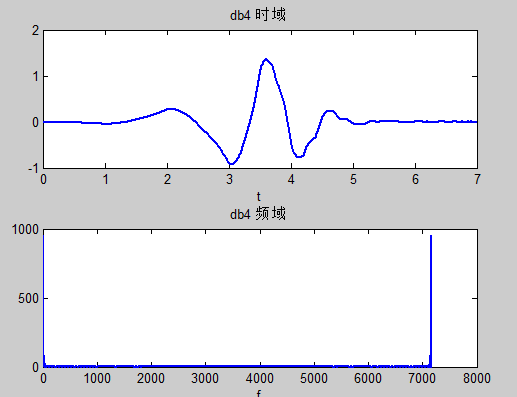Daubechies小波常用来分解和重构信号，作为滤波器使用:
[Lo_D,Hi_D,Lo_R,Hi_R] = wfilters('db4'); %计算该小波的4个滤波器
subplot(2,2,1);
stem(Lo_D,'LineWidth',2);
title('分解低通滤波器');
subplot(2,2,2);
stem(Hi_D,'LineWidth',2);
title('分解高通滤波器');
subplot(2,2,3);
stem(Lo_R,'LineWidth',2);
title('重构低通滤波器');
subplot(2,2,4);
stem(Hi_R,'LineWidth',2);
title('重构高通滤波器');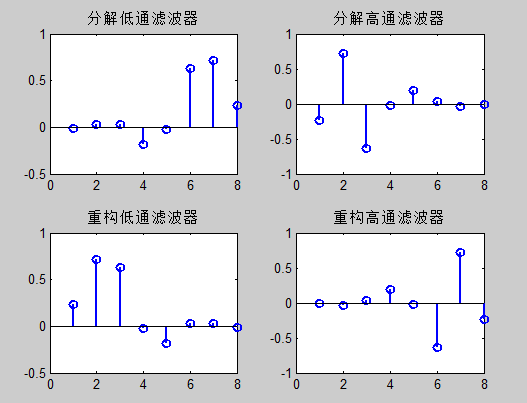3.Mexican Hat(mexh)小波Mexican Hat函数为Gauss函数的二阶导数：因为它的形状像墨西哥帽的截面，所以也称为墨西哥帽函数。Mexihat小波的时域和频域波形：

  Mexihat小波的时域和频域波形：
d=-6; h=6; n=100;
[g1,x]=mexihat(d,h,n);
subplot(2,1,1);
plot(x,g1,'LineWidth',2);
xlabel('t');
title('Mexihat 时域');
g2=fft(g1);
g3=(abs(g2));
subplot(2,1,2);
plot(g3,'LineWidth',2);
xlabel('f');
title('mexihat 频域');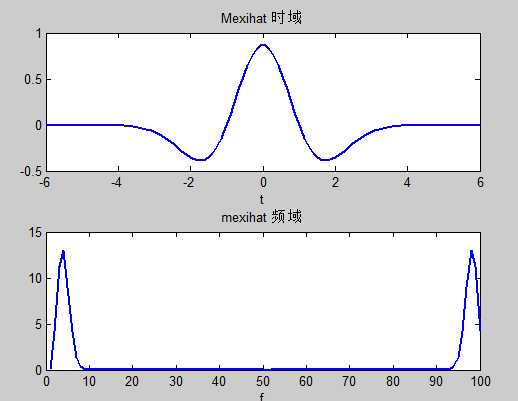Mexihat小波的特点：在时间域与频率域都有很好的局部化，并且满足。不存在尺度函数，所以Mexihat小波函数不具有正交性。

4.Morlet小波它是高斯包络下的单频率副正弦函数：其中C是重构时的归一化常数。Morlet小波没有尺度函数，而且是非正交分解。Morlet小波的时域和频域波形图：

  d=-6; h=6; n=100;
[g1,x]=morlet(d,h,n);
subplot(2,1,1);
plot(x,g1,'LineWidth',2);
xlabel('t');
title('morlet 时域');
g2=fft(g1);
g3=(abs(g2));
subplot(2,1,2);
plot(g3,'LineWidth',2);
xlabel('f');
title('mexihat 频域');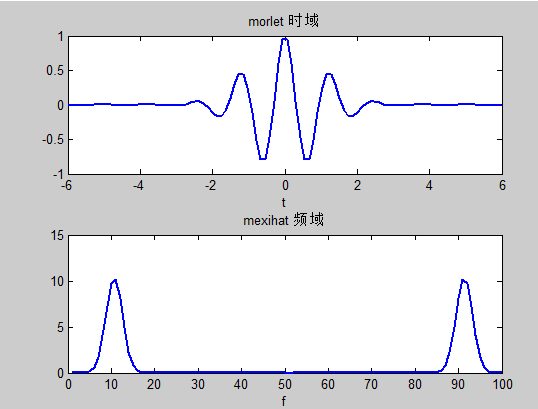注:

获取小波系数的两个函数理解：

Wpcoef 是求解某个节点的小波包系数，数据长度是1/(2^n)（分解n层的话），其实就是将原始信号化成2^N段，每段的长度是相等的且比原信号短

wprcoef是把某个节点的小波包系数重构，得到的是和原信号一样长度的信号。

傅里叶变换与小波变换理解参考:

https://zhuanlan.zhihu.com/p/22450818

https://zhuanlan.zhihu.com/p/19763358

https://www.jianshu.com/p/5b5c160c7e3a


展开全文• 参考： 小波与小波包、小波包分解与信号重构、小波包能量特征提取 暨 小波包分解后实现按频率大小分布重新排列(Matlab 程序详解）参考：

小波与小波包、小波包分解与信号重构、小波包能量特征提取 暨 小波包分解后实现按频率大小分布重新排列(Matlab 程序详解）


展开全文信号处理
• 这篇文章介绍了小波分解和小波包分解。小波分解(wavelet transform)小波傅里叶变换的基本方程是sin和cos，小波变换的基本方程是小波函数(basic wavelet)，不同的小波在波形上有较大的差异，相似的小波构成一个小波族...
这篇文章介绍了小波分解和小波包分解。小波分解(wavelet transform)小波傅里叶变换的基本方程是sin和cos，小波变换的基本方程是小波函数(basic wavelet)，不同的小波在波形上有较大的差异，相似的小波构成一个小波族(family)。小波具有这样的局部特性:只有在有限的区间内取值不为0。这个特性可以很好地用于表示带有尖锐， 不连续的信号。小波变换α=WTfα=WTf 其中αα 表示变换得到的小波系数，W是正交矩阵。ff 是输入信号。正交矩阵构造特定的小波函数(basic wavelet)由一组特定的小波滤波系数(wavelet filter coefficients)构成。当选定了小波函数，其对应的那组小波滤波器系数就知道。用小波滤波器系数构造不同维度的低通滤波器和高通滤波器(下面的例子中W就是由这些系数构造出来的)。低通滤波器可以看作为一个平滑滤波器(smoothing filter)。这两个滤波器，低通和高通滤波器，又分别被称为尺度(scaling)和小波滤波器(wavelet filter)。一旦定义好了这两个滤波器，通过递归分解算法(也称为金字塔算法(pyramid algorithm),树算法(tree algorithm)将得到水平多分辨率表示的信号。树算法原始信号通过低通滤波器得到低频系数 (approximate coefficients), 通过高通滤波器得到高频系数(detail coefficients)。把第一层的低频系数作为信号输入，又得到一组approximate coefficients和detail coefficients。再把得到的approximate coefficients作为信号输入，得到第二层的approximate coefficients和detail coefficients。以此类推，直到满足设定的分级等级。最大的分解等级为log2Nlog2N.用数学表达就是：原始信号可看做0级低频系数 a0=(f0,f1,...,fn)a0=(f0,f1,...,fn);那么am=G∗am−1am=G∗am−1, dm=Ham−1dm=Ham−1， G,H 分别表示低通滤波器和高通滤波器，用矩阵表示。信号的重构am−1=G∗am+H∗dmam−1=G∗am+H∗dm  G∗G∗,H∗H∗为G,H 的共轭矩阵。例子：使用Haar小波做离散小波变换Haar小波是最简单的小波函数。归一化的小波滤波器系数只有两个c0=12−−√=0.7071c0=12=0.7071, c1=−0.7071c1=−0.7071. 低通滤波器由0.7071，0.7071组成，高通滤波器由0.7071，-0.7071组成，用矩阵G和H表示，矩阵的维度由信号的长度决定。分解的结果小波包分解(wavelet packet transform)简单理解就是每一层分解得到的系数都要再分解，不像小波分解那样只有低频系数会再分解。同样以Haar小波为例子。分解结果展开全文• 基于奇异值分解和小波包分解的故障检测李一博，沈慧，高远【摘要】根据真空泵在故障和正常模式下工作时，其振动信号在频域的能量分布的差异性，设计基于奇异值分解(SVD)和小波包分解(WPD)的真空泵故障检测方法。...
基于奇异值分解和小波包分解的故障检测李一博，沈慧，高远【摘要】根据真空泵在故障和正常模式下工作时，其振动信号在频域的能量分布的差异性，设计基于奇异值分解(SVD)和小波包分解(WPD)的真空泵故障检测方法。首先用SVD对采集到的信号进行去噪，再使用小波包对去噪后的信号进行分解，对分解得到的各层系数进行重构并提取需要的各频域段的能量。将提取的能量向量作为支持向量机(SVM)的输入样本，对SVM进行训练。最后使用实验数据对SVM的可靠性进行验证。实验结果表明，采用SVD和WPD结合的方法能较好地识别出真空泵的故障。【期刊名称】电子技术应用【年(卷),期】2018(044)003【总页数】4【关键词】真空泵故障；奇异值分解；小波包分解；支持向量机0引言目前，随着我国航空航天科学技术的发展，尤其是空间推进技术的大力发展，航天器空间模拟实验对实验设备的要求正在逐步提高。而超低温和高真空是模拟实验所重点要求的重要的测试环境。其中，真空泵是空间模拟器的核心设备之一，真空泵能否正常工作，将决定空间环境模拟器能否正常有效地完成航天器的真空热环境实验。其次，中国拥有大量的航空航天基地，还有冶金行业等，真空泵的持有数量巨大。因而，无论是从设备安全角度还是从社会经济利益出发，对真空泵运行故障进行检测都具有重要的意义。在传统的机械故障诊断技术中，傅里叶变换是最常用的频域信号处理方法，但
展开全文• 小波与小波包、小波包分解与信号重构、小波包能量特征提取小波与小波包区别小波分解小波包分解小波包——小波包树与时频图小波包树解读：Matlab实例时间频率图主要解释小波包-----小波包分解系数小波包-----信号分解...信号处理 matlab
• ## 小波分解和小波包分解

万次阅读 多人点赞 2018-03-04 16:39:53
这篇文章介绍了小波分解和小波包分解。 小波分解（wavelet transform） 小波 傅里叶变换的基本方程是sin和cos，小波变换的基本方程是小波函数(basic wavelet)，不同的小波在波形上有较大的差异，相似的小波...小波变换
• 输入原始数据和小波包参数，输出小波包分解后的数据并画图
• 利用labview编程实现信号的小波包分解，并克服了传统小波包分解频编码混乱的问题，同时实现了功率谱以及频带能量的分析
• ## 小波包分解程序

热门讨论 2013-10-29 18:21:18
• 对信号序列进行小波包分解，获取没层分解的归一化能量，并绘制能量谱图
• clear;clc;n=3;wpname=’db3’;% [b,a]=butter(8,[5/100 99/100]);% load a1_1-1;load k301_1-4;% Data=filter(b,a,Data);Data=Data-mean(Data);Data=detrend(Data);... %对数据进行小波包分解for i=1:2^...
• 小波分解广泛应用于信号处理，在计算机图像信号处理中，图像重构技术较为关键，小波包分解可对图像信号各个频段进行及其细化的划分。文中分别对图像处理，小波分解，小波包分解进行阐述，并应用MATLAB对二维小波包...
• MATLAB 小波包分解遇到的问题 最近已经初步完成电机轴承故障单点故障的诊断，想紧接着做下电机轴承复合故障的诊断。在查找文献时发现有一种比较合适的方法，先是对加速度传感器获得的振动信号进行小波包分解，这个...
• 通过Matlab对信号进行小波包分解处理，代替常用心理声学模型的FFT，改善了时频分辨率不足的问题，且通过小波包分解得到的频带划分，比常用心理声学模型得到的频带划分更接近于人耳的临界频带，更适应于人耳的听觉...
• 图1为用MATLAB绘制的小波包分解树，分解层数为3层。树中节点的命名规则如下：从（1,0）开始，（1,0）为1号，（1,1）是2号，依次类推，（3,0）是7号，（3,7）是14号。每个节点都有对应的小波包系数，此系数决定了频率...
•研究论文
•研究论文
• 根据真空泵在故障和正常模式下工作时，其振动信号在频域的能量分布的差异性，设计基于奇异值分解(SVD)和小波包分解(WPD)的真空泵故障检测方法。首先用SVD对采集到的信号进行去噪，再使用小波包对去噪后的信号进行...
•研究论文
• 对一幅图像的进行小波包分解，然后提取每一层的系数
• matlab开发-特征提取使用多信号小波包分解。它只是一个使用小波包变换（WPT）的特征提取代码。...